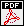## Equivariant epsilon conjecture for 1-dimensional Lubin-Tate groups

Autors: Dmitriy Izychev and Otmar Venjakob
Titel:  Equivariant epsilon conjecture for 1-dimensional Lubin-Tate groups
Jahr: 2013
Seiten: 21
In: J. Théor. Nombres Bordeaux 28 (2016), no. 2, 485--521.Abstract: In this paper we formulate a conjecture on the relationship between the equivariant \epsilon-constants (associated to a local p-adic representation V and a finite extension of local fields L/K) and local Galois cohomology groups of a Galois stable \mathbb{Z}_{p}-lattice T of V. We prove the conjecture for L/K being an unramified extension of degree prime to p and T being a p-adic Tate module of a one-dimensional Lubin-Tate group defined over \mathbb{Z}_{p} by extending the ideas of \cite{Breu} from the case of the multiplicative group \mathbb{G}_{m} to arbitrary one-dimensional Lubin-Tate groups. For the connection to the different formulations of the \epsilon-conjecture in \cite{BB}, \cite{FK}, \cite{Breu}, \cite{BlB} and \cite{BF} see \cite{Iz}.